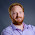## Monday, 17 September 2012

### Order of operations

I was recently asked about the order of operations (or "operator precedence") in Uniface, and I couldn't really answer the question with an certainty, so I thought I'd investigate and prove or disprove my assumptions.  It relates primarily to mathematical expressions, but also to logical conditions, and defines in what order the various components are processed.

Take for example the following mathematical expression...

x = 10 * 5 + 1

Operator precedence states that multiplication is done before addition, therefore x is 51, not 60.

Wikipedia states that the following order of operations should be true for most programming languages, so my question is, does Uniface comply?

 1 ()   []   ->   .   :: Grouping, scope, array/member access 2 !   ~   -   +   *   &   sizeof   type cast ++x   --x (most) unary operations, sizeof and type casts 3 *   /   % Multiplication, division, modulo 4 +   - Addition and subtraction 5 <<   >> Bitwise shift left and right 6 <   <=   >   >= Comparisons: less-than, ... 7 ==   != Comparisons: equal and not equal 8 & Bitwise AND 9 ^ Bitwise exclusive OR 10 | Bitwise inclusive (normal) OR 11 && Logical AND 12 || Logical OR 13 ?: Conditional expression (ternary operator) 14 =   +=   -=   *=   /=   %=   &=   |=   ^=   <<=   >>= Assignment operators 15 , Comma operator

I think it's going to be easiest to prove these in reverse order, so let's take them one by one...

15 - Comma operator

Uniface doesn't have comma operators, you cannot put multiple lines of code on the same line with any separator, as far as I'm aware.

14 - Assignment operators

I wrote a previous post on assignment operators, the arithmetic ones (+= -= *= /= %=) all work in Uniface, but the logical ones (&= |= ^= <<= >>=) do not.  The simplest assignment operator (=) is also used as the comparison operator, there is no differentiation in Uniface.  This means that the comparison operator takes precedence, which be proved with a simple if statement...

x = 0
if ( x = 1 )
;if assignment took precedence
endif

13 - Conditional expression

This is not valid syntax in Uniface either, you have to do it the long way.  I never really liked this syntax in javascript though anyway, less readable in my opinion.

11/12 - Logical and/or

The logical operators in Uniface are singular (& and |), although the double versions (&& and ||) also work the same way, as I discovered in an earlier post.  I tried to combine these with the assignment operators in order to determine the precedence, but all I got was compile errors.  It seems that assignment operators cannot be used inline.

8/9/10 - Bitwise and/xor/or

Uniface does not have bitwise operators, as previously discussed.  The singular "and" and "or" operations are the logical operators.

6/7 - Comparison

As mentioned above, the equals comparison operation (=) is the same as the simplest assignment operator, and the comparison takes precedence.  It also takes precedence over the logical operators, as this if statement proves...

x = 0
if ( x = 1 | 1)
x = 1 ;if ((x = 1) | 1)
else
x = 0 ;if (x = (1 | 1))
endif

5 - Bitwise shift

Uniface still doesn't have bitwise operators :)

It's simple enough to show that the addition (+) and subtraction (-) operators take precedence over the comparison operator (=), using another if statement...

x = 0
if ( x = 0 + 1 )
x = 1 ;if (x = (0 + 1))
else
x = 0 ;if ((x = 0) + 1)
endif

3 - Multiplication/division/modulo

It's also simple to show that the multiplication (*), division (/) and modulo (%) operators take precedence over the addition and subtraction operators...

x = 6 * 1 - 1
;(6 * 1) - 1 = 5
;6 * (1 - 1) = 0

2 - Unary/type cast

Uniface doesn't support all the unary operators, in fact I'm not sure what all of them do.  The not (!) operand is easy enough to test though...

x = ! 0 + 1
;(! 0) + 1 = 2
;! (0 + 1) = 0
askmess "x=%%x%%%" ;shows "x=0" but would have expected "x=2"

Surprisingly this one comes up with the opposite result than was expecting, indicating that addition (+) actually takes precedence over not (!).  We know that Uniface is good at type casting though, and will always try to cast a string as a numeric when doing arithmetic operations...

x = "1" * 2

We can also show the negative unary operator (-) takes precedence over the subtraction operator (-), despite these being the same, like this...

x = -1 - 2
;(-1) - 2 = -3
;-(1 - 2) = 1

1 - Grouping

Uniface doesn't have arrays or members, and scope is handled explicitly with end statements.  It does however use brackets for grouping, as we've seen through these examples, they can be used to change the order of precedence, as they are always done first.

Summary: It seems that Uniface is a relatively simple in the sense that it doesn't do complicated inline nesting, nor any bitwise operations.  With the exception of the not operator (!), it seems to follow the standard order of operations though.  Personally I prefer to use lots of brackets in order to make the order of precedence more obvious and easier to read, without having to remember these rules.

#### 1 comment:

1.Uniface refers to assignment operators (14) as "compound operators", I discovered today!